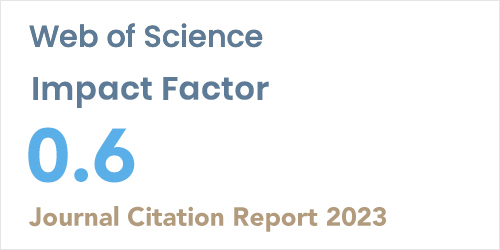ISSN: 1304-7191 | E-ISSN: 1304-7205
Volume: 41 Issue: 5 Year: 2023Analysis of Grover’s quantum search algorithm on a classical computer: Identifying opportunities for improvement
1Gümüşhane University, Department of Physics Eng., Gumushane, Turkey
2Gümüşhane University, Department of Software Eng., Gumushane, Turkey
Sigma J Eng Nat Sci - DOI: 10.14744/sigma.2023.00069

#### Abstract

In this paper, Grover’s quantum search algorithm is analyzed using a classical computer by calculating the amplitudes and the probabilities of finding a single marked state for n=5, 10, 15, 20, 25, and 27 qubit states. The calculations show that the marked state can be found in O(√N) iterations, where N=2^n is the number of items. The possibility of improving Grover’s search algorithm to find a single item in N search elements is discussed by calculating the amplitudes and hence the probabilities of finding a single marked state for n=5, 10, 15, 20, 25, 30, 35, 40, 45, and 50 qubit states. The calculations showed that the marked state could be found with sufficiently high probability in O(ln⁡(N)) iterations. This is quite a remarkable speed-up that can be achieved to find a single marked element in an unsorted N search element.